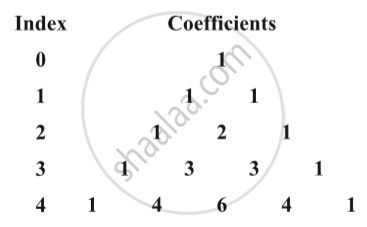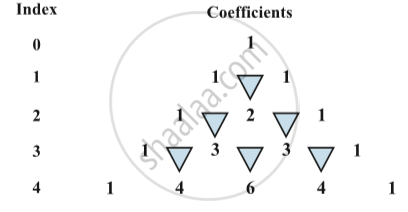Advertisement Remove all ads

# Binomial Theorem for Positive Integral Indices

Advertisement Remove all ads

#### description

• Statement and Proof of the Binomial Theorem for Positive Integral Indices
• Proof of Binomial Therom by Induction
• Special Case in Binomial Therom
• Pascal's Triangle
• Binomial theorem for any positive integer n
• Some special cases-(In the expansion of (a + b)n)

#### notes

Let us have a look at the following identities done earlier:
(a+ b)^0 = 1
a + b ≠ 0
(a+ b)^1 = a + b
(a+ b)^2 = a^2 + 2ab + b^2
In these expansions, we observe that
(i) The total number of terms in the expansion is one more than the index. For example, in the expansion of (a + b)^2 , number of terms is 3 whereas the index of (a + b)^2 is 2.
(ii) Powers of the first quantity ‘a’ go on decreasing by 1 whereas the powers of the second quantity ‘b’ increase by 1, in the successive terms.
(iii) In each term of the expansion, the sum of the indices of a and b is the same and is equal to the index of a + b.
We now arrange the coefficients in these expansions as following fig.The addition of 1’s in the row for index 1 gives rise to 2 in the row for index 2. The addition of 1, 2 and 2, 1 in the row for index 2, gives rise to 3 and 3 in the row for index 3 and so on. Also, 1 is present at the beginning and at the end of each row. This can be continued till any index of our interest.

Pascal's triangle:The structure given in Fig.  looks like a triangle with 1 at the top vertex and running down the two slanting sides. This array of numbers is known as Pascal’s triangle, after the name of French mathematician Blaise Pascal. It is also known as Meru Prastara by Pingla.
For this, we make use of the concept of combinations studied earlier to rewrite
the numbers in the Pascal’s triangle. We know that
"^nC_r = (n!)/(r!(n-r))! , 0 <= r <= n

If you would like to contribute notes or other learning material, please submit them using the button below.

### Shaalaa.com

Binomial Theorem for Positive Integral Indices [00:08:59]
S
##### Series:
0%

Advertisement Remove all ads
Share
Notifications

View all notifications

Forgot password?